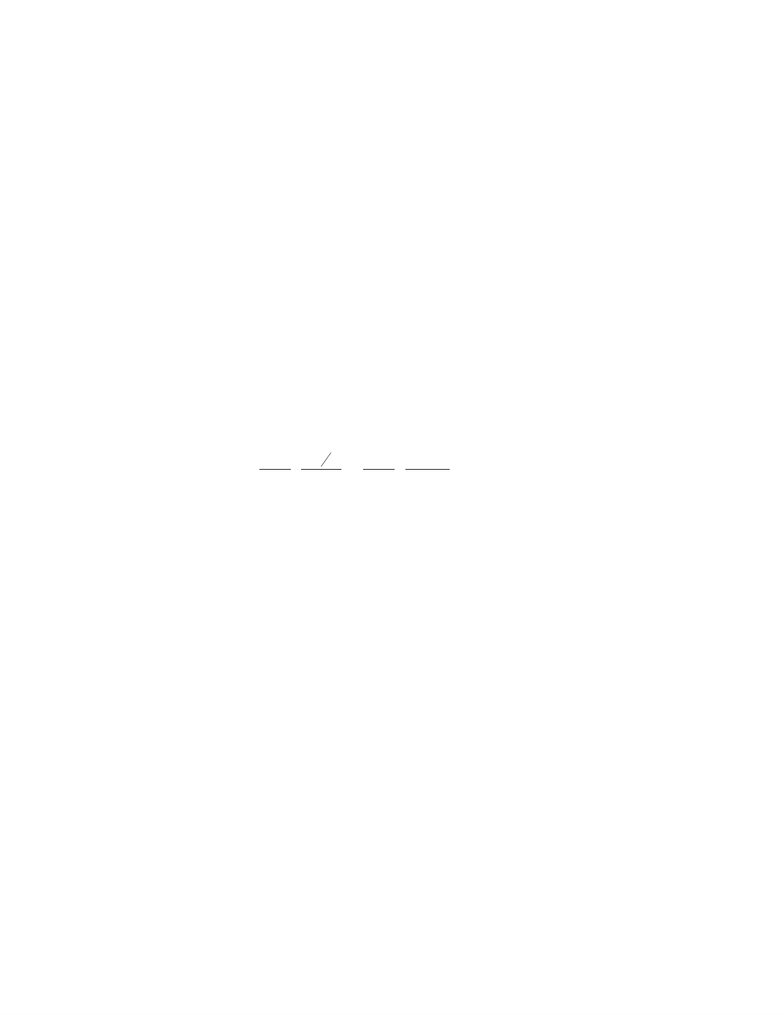Study Guides (400,000)
CA (160,000)
Ryerson (10,000)
Finance (300)
FIN 300 (100)

# Technical Note - Solved Capita Budgeting Problems.doc

Department
Finance
Course Code
FIN 300
Professor
Michael Inglis

This preview shows page 1. to view the full 4 pages of the document.Technical Note Solved Capital Budgeting Problems
General Points:
Remember that capital budgeting problems are based on cash flow from assets which
is composed of three types of cash flows; 1) capital expenditure / sales, 2) operating
cash flows and, 3) changes in NWC. We will be using the CCA tax shield formula to
solve all of these problems so we will write the operating cash flow as:
OCF = (S-C)(1-T) + DT
The first term, (S-C)(1-T) is the after tax operating cash flow but ignoring the impact
of depreciation. Remember depreciation (i.e. CCA) in and of itself is not important
(it’s a non cash item) however it does reduce your taxes. The second term, DT, is the
CCA tax shield which represents the dollar reduction in your taxes, in a given year,
due to the ability to deduct CCA before calculating your taxable income. We don’t
want to work out all of the individual CCA or tax shield numbers for each year of a
project so we will use the CCA tax shield formula to find the PV of all future tax
shields in one fell swoop.
12
11
() 1 (1 )
:
capital cost
d = CCA rate
T = corp tax rate
r = cost of capital
S = salvage value
n = life of project
n
CdT r SdT
PV CCATS r d r r d r
where
C





 
 
If we use this formula we will not explicitly estimate the DT terms for each year so
all we have to worry about is the (S-C)(1-T) component of operating cash flow.
1) Replacement Problem
A firm is considering the purchase of a new machine, priced at \$350,000 to replace an
older machine. The economic life of the machine is 8 years at which time the
estimated salvage value is \$85,000. The present market value of the older machine is
\$50,000 and is expected to have a salvage value of \$15,000 in 8 years. The new
machine is expected to reduce operating expenses by \$50,000 per year. The firm’s
tax rate is 40% and its cost of capital is 15%. Both machines belong to class 10 with
a CCA rate of 30%. Should the firm replace its older machine?